# GMAT Math : Rate Problems

## Example Questions

← Previous 1

### Example Question #1 : Rate Problems

Data sufficiency question- do not actually solve the question

How many hours did it take to drive from city A to city B without stopping?

1. The drive started at 10 am.

2. The average speed during the trip is 65 miles/hour.

Statement 2 is sufficient, but statement 1 is not sufficient to answer the question

Statements 1 and 2 are not sufficient, and additional data is needed to answer the question

Both statements taken together are sufficient to answer the question but neither statement alone is sufficient

Each statement alone is sufficient to answer the question

Statement 1 is sufficient, but statement 2 is not sufficient to answer the question

Statements 1 and 2 are not sufficient, and additional data is needed to answer the question

Explanation:

The total time is calculated by the equation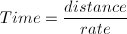. Statement 2 provides the rate, but we have no information regarding distance, therefore, the quesiton is impossible to solve without more information.

### Example Question #2 : Rate Problems

The route from Tom's house to his mother's house involves traveling for 55 miles along Interstate 10, then 20 miles along State Route 34. How long does it take for Tom to travel between the two houses, if he drives at precisely the speed limit on each highway?

Statement 1: The speed limit on Interstate 10 is 70 miles per hour for the entire stretch.

Statement 2: The speed limit on State Route 34 is 50 miles per hour for the first half of the distance Tom drives on it, and 40 miles per hour for the second half.

Statement 2 ALONE is sufficient to answer the question, but Statement 1 ALONE is NOT sufficient to answer the question.

BOTH statements TOGETHER are insufficient to answer the question.

EITHER statement ALONE is sufficient to answer the question.

Statement 1 ALONE is sufficient to answer the question, but Statement 2 ALONE is NOT sufficient to answer the question.

BOTH statements TOGETHER are sufficient to answer the question, but NEITHER statement ALONE is sufficient to answer the question.

BOTH statements TOGETHER are sufficient to answer the question, but NEITHER statement ALONE is sufficient to answer the question.

Explanation:

The rate formula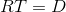- or, actually, the equivalent equation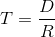- will help.

Let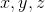be the speed limits along the interstate, the first half of the state route, and the second half of the state route, respectively.

Then Tom can drive I-10 in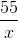hours; the first half of SR 34,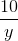; the second half of SR 34,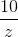.

The timeit takes, in hours, for Tom to make the entire trip is the sum of these fractions: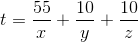.

To calculate, we need. Statement 1 only tells us; Statement 2 only tells usand. Therefore, both together, but neither alone, are enough to allow us to calculate.

### Example Question #3 : Rate Problems

Cassie and Derek must decorate 60 cupcakes. It takes them 150 minutes to complete the task together. How long would it take Derek to decorate the cupcakes by himself?

Statement 1: It would take Cassie 240 minutes to decorate the cupcakes by herself.

Statement 2: Derek can decorate 9 cupcakes in 1 hour.

Each statement alone is sufficient.

Both statements taken together are sufficient to answer the question, but neither statement alone is sufficient.

Statement 1 alone is sufficient, but Statement 2 alone is not sufficient to answer the question.

Statements 1 and 2 together are not sufficient to answer the question.

Statement 2 alone is sufficient, but Statement 1 alone is not sufficient to answer the question.

Each statement alone is sufficient.

Explanation:

From the first statement, we can calculate the number of cupcakes Cassie can decorate in 150 minutes. From there, we can calculate the rate at which Derek decorates. Therefore, Statement 1 alone is sufficient to answer the question.

The second statement gives the rate at which Derek decorates cupcakes. Therefore, Statement 2 alone is also sufficient to answer the question.

### Example Question #1 : Rate Problems

Two trains (Train A and Train B) leave their stations at exactly 6 pm, travelling towards each other from stations exactly 60 miles apart. There are no other stops between these two stations. What time does Train B arrive at its destination?

1. Train A travels twice as fast as Train B.

2. At 6:40 pm, the two trains pass each other.

Statement 2 alone is sufficient, but Statement 1 alone is not sufficient to answer the question.

Statements 1 and 2 together are not sufficient.

Both statements taken together are sufficient to answer the question, but neither statement alone is sufficient.

Statement 1 alone is sufficient, but Statement 2 alone is not sufficient to answer the question.

Each statement alone is sufficient.

Both statements taken together are sufficient to answer the question, but neither statement alone is sufficient.

Explanation:

Statement 1 alone does not tell us enough about the speed of train B to answer the question. Statement 2 alone does not tell us about the rates of either individual train, so it is also not sufficient by itself to answer the question.

But can we figure out the answer using both pieces of information? Yes, we can! If we know that the trains meet at 6:40, then their combined rate of travel is 60 total miles in 40 minutes. Statement 1 says that Train A travels twice as fast as Train B, so we can determine the distances covered by the two trains in those 40 minutes. From there, we can find rates for both trains and then answer the question. Thus, both statements together are sufficient to answer the question, but neither statement alone is sufficient.

Note: We didn't actually answer the question of Train B's arrival time. For data sufficiency questions, don't waste time trying to find the specific answer. All that is necessary is determining whether or not it is POSSIBLE to answer the question with the information given.

### Example Question #31 : Data Sufficiency Questions

Jake and Colin are painting a house together. How long would it take Colin to paint the house alone?

(1) Jake and Colin can paint the house together in 6 hours, working at the same rate.

(2) Jake can paint the house alone in 12 hours

Statement (2) alone is sufficient.

Statements (1) and (2) are insufficient.

Statement (1) alone is sufficient.

Each statement alone is sufficient.

Both statements taken together are sufficient.

Statement (1) alone is sufficient.

Explanation:

Here we can see that there are two people painting the house, so we should set the following equation :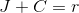, where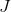is the rate for Jake and whereis the rate for Colin,stands for their rate together. Statement one gives us a value forand even though it doesn't tell us what isorit tells us that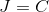which allow us to answer the question.

For Statement 2, we are left with two other unknowns in our equation and we can clearly see that (2) is not sufficient.

### Example Question #1 : Rate Problems

Celine and Jack are painting a house together at their respective rates. After working 1 hour together, Jack starts to feel tired and stops. How long does it take Celine to finish the work?

(1) Celine could have painted the house alone in 3 hours.

(2) It would have taken Jack 5 hours to paint the house alone.

Statements (1) and (2) taken together are not sufficient.

Both statements taken together are sufficient.

Each statement alone is sufficient.

Statement (2) alone is sufficient

Statement (1) alone is sufficient.

Both statements taken together are sufficient.

Explanation:

We should start by setting equationsand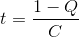, these are the two equation we should be able to solve. Where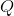is the amount of the work they did togetherm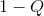is the amount of work left,is Celine's rate andis the rate of Celine and Jack together. So withwe can see that we would need both Jack and Celine's rate. Since they work at a different rate, we shoud only be able to answer this problem taking both statements together.

### Example Question #1 : Rate Problems

Machine X and machine Y both produce screwdrives at their respective rates. What is the rate of the machines working together?

(1) Machine X can produce 200 screwdrivers in an hour and machine Y can produce as many in 2 more hours.

(2) Machine Y can produce 200 screwdrivers in 3 hours. Machine X is three times as fast as machine Y.

Statement (1) alone is sufficient.

Statements (1) and (2) are not sufficient.

Each statement alone is sufficient.

Both statements taken together are sufficient.

Statement (2) alone is sufficient.

Each statement alone is sufficient.

Explanation:

To be able to solve this problem, we need to figure out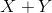whereis the rate of machine X and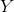is the rate of machine Y. Statement 1 tells us the rate for machine X, but tells us as well that it takes 2 more hours for machine Y to produce the same amount of screwdrivers. Therefore, we are told that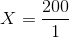and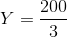, which is sufficient to answer the question. Statement 2 tells us that the rate of machine Y isand also that that rate of machine X is 3 times as fast as machine Y: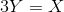soEach statement alone is therefore sufficient.

### Example Question #2 : Dsq: Calculating Rate

Machine O working together with machine P produces telephones at a rate of 1056 per hour. How many telephones can machine O produce in an hour?

(1) It takes machine P four hours longer than machine O to produce 880 telephones.

(2) Machine P produces telephones at a rate of 176 telephones per hour.

Both statements together are sufficient.

Statement (1) alone is sufficient.

Each statement alone is sufficient.

Statements 1 and 2 together are not sufficient.

Statement (2) alone is sufficient.

Each statement alone is sufficient.

Explanation:

To solve this problem we first have to set up an equation for our variables: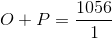where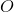is the rate of machine O andis the rate of machine P.

The first statement tells us that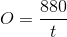and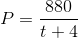, whereis the time it takes machine O to produce 880 telephones.

At first it might look insufficient but, by pluging in the values forandin our first equation we get :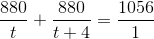, this gives us a quadratic equation, in which we can solve and find t, and therefore find the number of telephones O can produce in 1 hour.

The second statement tell us that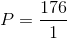, therefore, we can plug in this value in our first equation to find the rate for machine O and this will allow us to answer the question.

### Example Question #1 : Rate Problems

Machines A,B and C working together take 12 minutes to complete a watch.

What is the rate of both machines B and C working together?

(1) Machines A and B together can complete a watch in 20 minutes.

(2) Machines A and C together can complete a watch in 15 minutes.

Statement (1) alone is sufficient.

Both statements taken together are sufficient.

Each statement alone is sufficient.

Statements (1) and (2) taken together are not sufficient.

Statement (2) alone is sufficient.

Both statements taken together are sufficient.

Explanation:

Firstly we should set up an equation for this problem: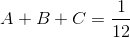where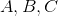are the respective rates of machines A, B and C. We need to find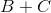.

Statement 1 tells us that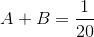.

This alone is not suffient since we will have no precise information on machine B. Similarly, with statement 2 We can find the value of the rate for machine B but we cannot know what is the rate of machine C, from what we are told. However, taking both statements together, with statement 1 we can find the value forand with statement 2 we can find the value forand thereby we can find the value of.

### Example Question #31 : Data Sufficiency Questions

16 very precise machines, working at the same constant rate, can produce a luxury necklace working together. How many machines should we add in order for the necklace to be produced in an hour.

(1) It takes 5 hours for these machines to produce the necklace

(2) A single machine produces a necklace in 80 hours.

Both statements together are sufficient.

Each statement alone is sufficient.

Statement (2) alone is sufficient.

Statement (1) alone is sufficient.

Statements 1 and 2 together are not sufficient.

Each statement alone is sufficient.

Explanation:

We know that there are 16 machines and we are looking for how many we should add. Let us set an equation representing the number of machines in use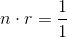, whereis the total number of machines we need andis the rate of an individual machine. We should therefore find a rate for a single machine to be able to solve this problem, since we can then calculate.

Statement 1 tells us that for 16 machines, it takes 5 hours to produce a necklace. From this we can find the rate of a single machine.

Statement 2 tells us direclty the rate of a single machine, therefore both these statements allow us to answer the problem.

← Previous 1

### All GMAT Math Resources